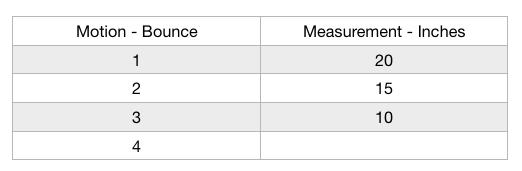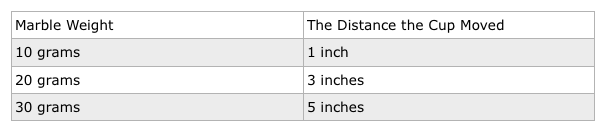3rd Grade Science : Make measurements of an object's motion

Example Questions

Example Question #1 : Make Measurements Of An Object's Motion

Ashley wants to investigate how objects move and see if she can make correct predictions. She knows she will have to observe the object's motion. She wants to see which surface material will allow a toy car to get down a ramp in the least amount of time.

What will Ashley measure to determine which car was the fastest?

Ashley will measure the speed of the car as it exits the ramp.

Ashley will measure the time it takes for each car to go down the ramp.

Ashley will measure the weight of each material on the ramp.

Ashley will measure the distance the car traveled on the ramp.

Ashley will measure the time it takes for each car to go down the ramp.

Explanation:

Ashley will be investigating which car gets down the ramp in the least amount of time. The keyword "time" in that statement gives a clue to which answer choice would be the correct measurement. The only answer choice that measures time is, "Ashley will measure the time it takes for each car to go down the ramp.". This choice would collect information about each car so their times can be compared. The vehicle with the least amount of time would be the car that travels down the ramp the fastest.

Example Question #2 : Make Measurements Of An Object's Motion

Cindy rolls a marble down a ramp and hits a cup waiting on the bottom. She does this multiple times with different size marbles. After each roll, she measures the distance the cup traveled.

Which tool would be best for measuring the object's movement?

A ruler

A meter stick

A measuring cup

A balance

A ruler

Explanation:

The tool that would be most appropriate for measuring would be a ruler. This investigation is measuring distance so that would narrow the choices down to a ruler or the meter stick. A meter stick measures things that are a longer-distance, and a marble is not going to move a cup that far. A ruler would be sufficient for this investigation.

Example Question #3 : Make Measurements Of An Object's Motion

Jamarcus is experimenting at his local playground. He and his friends are all wearing pants made of different materials. They want to see which type of pants will move down the slide quickest.

Which tool should they use to collect this data?

A stopwatch

A pen and paper

A scale

A ruler

A stopwatch

Explanation:

This experiment is testing which pants' material will slide the fastest. This is a measurement of time and speed. The tool that would be the most appropriate would be the stopwatch. The boys could time each person from the top of the slide to the bottom to determine who's pants help them to slide the fastest. The person with the lowest time is the material that allows the quickest slide.

Example Question #4 : Make Measurements Of An Object's Motion

Which tool would be most appropriate for measuring how high the ball bounces in this experiment? Use the data table below for additional information.A yardstick

A scale

A balance

A stopwatch

A yardstick

Explanation:

In this scenario, the students measure distance, and they measure up to 20 inches. A yardstick would be most appropriate because it uses the correct units of measurement and would reach the necessary length to complete the measurements.

Example Question #5 : Make Measurements Of An Object's Motion

What would be the most appropriate tool to measure the weight or mass of the marbles in the data table below?A beaker

A measuring cup

A ruler

A scale

A scale

Explanation:

This data table shows the marble's weight is in grams. To measure the marble accurately, a scale should be used to find its weight or mass. A beaker and measuring cup would be more appropriate for liquids, and a ruler measures length. A scale or balance would be the best option for weight or mass.

Example Question #6 : Make Measurements Of An Object's Motion

Grant wants to investigate how objects move and see if he can make correct predictions. He knows he will have to observe the object's motion. He wants to see if a soccer ball will travel further on artificial turf or real grass.

What will Grant need to measure to determine which surface the soccer ball travels furthest on?

Grant will measure the distance the balls traveled on each type of grass.

Grant will measure the speed of the ball as it moves across the grass.

Grant will measure the weight of each type of grass.

Grant will measure the time it takes for each ball to go across the grass.

Grant will measure the distance the balls traveled on each type of grass.

Explanation:

Grant will be investigating whether a soccer ball travels further on artificial turf or real grass. The keyword "further" in that statement gives a clue to which answer choice would be the correct measurement. The only answer choice that measures distance is, "Grant will measure the distance the balls traveled on each type of grass.". This choice would collect information about each grass type and the distance the balls traveled so they can be compared.

Example Question #7 : Make Measurements Of An Object's Motion

Jamarcus is experimenting at his local playground. He and his friends are all wearing pants made of different materials. They want to see which type of pants will move down the slide quickest.

What would be the best way to take measurements and determine which pants slide the fastest?

Jamarcus and his friends should start timing each person as they begin sliding to when they reach the bottom. They should record the measurements and then compare them to see which material had the shortest time.

Jamarcus and his friends should measure the distance from the top of the slide to the bottom of the slide. They should record the measurement and then determine which person is the shortest.

Jamarcus and his friends should measure the distance from the top of the slide to the bottom of the slide. They should record the measurement and then determine which person is the tallest.

Jamarcus and his friends should start timing each person as they begin sliding to when they reach the bottom. They should record the measurements and then compare them to see which material had the longest time.

Jamarcus and his friends should start timing each person as they begin sliding to when they reach the bottom. They should record the measurements and then compare them to see which material had the shortest time.

Explanation:

This experiment is testing which pants' material will slide the fastest. This is a measurement of time and speed. The boys could time each person from the top of the slide to the bottom to determine who's pants help them to slide the fastest. The person with the lowest time is the material that allows the quickest slide.

Example Question #8 : Make Measurements Of An Object's Motion

If a student wants to observe and measure an object's motion, he can take measurements of the object's _______________.

distance traveled

None of the answer choices are correct.

All of the answer choices are correct.

time

speed

All of the answer choices are correct.

Explanation:

If the student wishes to make observations about an object's motion, taking measurements is an excellent way to record the data and draw conclusions. The student could measure the speed of the objects, its distance traveled, or the amount of time it was moving. All of these answer choices are appropriate measurements.

Example Question #9 : Make Measurements Of An Object's Motion

There is no way to measure an object's motion. When something is moving, it cannot be measured.

True

False

False

Explanation:

The statement in the question is false. Objects can be measured when they are in motion. The object's speed is one example of a measurement that can be taken while the object is moving. Think about a baseball game, they record the speed of the ball and display it on the screen every time a pitcher throws the ball. In car races, the speed of the cars is measured to determine how fast they are traveling.

Example Question #10 : Make Measurements Of An Object's Motion

Cindy rolls a marble down a ramp and hits a cup waiting on the bottom. She does this multiple times with different size marbles. After each roll, she measures the distance the cup traveled.

What would be the best unit of measurement to use when she records her data?

Millimeters

Miles

Pounds

Inches